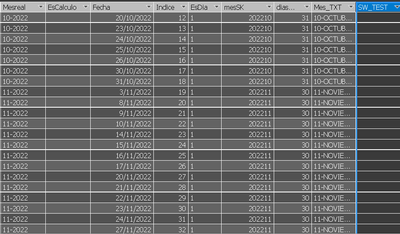cancel
Showing results for
Did you mean:Anonymous
Not applicable

## Create Calculated Column based on the result of a measure

Hello!
I need to create a calculated column that places a text depending on whether the condition of a measure is met

This is my table where I must create the Calculated Column:I require that if the Field [MesSK] is equal to the value of the measure [Mes0] I put "Current Month" if the value of the field [MesSK] is equal to the value of the measure [Mes1] I put "Previous Month", if the [MonthSK] field is equal to the value of the measure [Mes2] I put "Same month of the previous year", if the condition is not met I put "Not Applicable"

The measures are constructed in this way:

Mes0 := YEAR([Current Date])&FORMAT([Current Date];"mm")
Mes1:=var max_date = VALUE([Month0])
return CALCULATE([Mes0];FILTER(ALLSELECTED('DIM TIME');VALUE('DIM TIME'[mesSK])<max_date))
Mes2:=[Mes0]-100The calculated column will be used to create a line chart visual in the Legend field on the X axis going to the number of DaysBeforehand thank you very muchSuper User

@Anonymous , You should create a measure based on the measure. A calculated column can not take slicer values so no benefit

you can have row level measure

sumx(Table, [Measure]/[Column1])

or need Dynamic segmentation

Number - Dynamic segmentation -Measure to Dimension conversion: https://youtu.be/gzY40NWJpWQ

Text -Dynamic Segmentation, New/Lost/Retain Customer Count:
Learn Power BI Advance - Customer Retention with Dynamic Segmentation, New/Lost/Retain Customer Count: https://www.youtube.com/watch?v=W4EF1f_k6iY

Range Based

Dynamic Segmentation Bucketing Binning
https://community.powerbi.com/t5/Quick-Measures-Gallery/Dynamic-Segmentation-Bucketing-Binning/m-p/1387187#M626

Dynamic Segmentation, Bucketing or Binning: https://youtu.be/CuczXPj0N-k